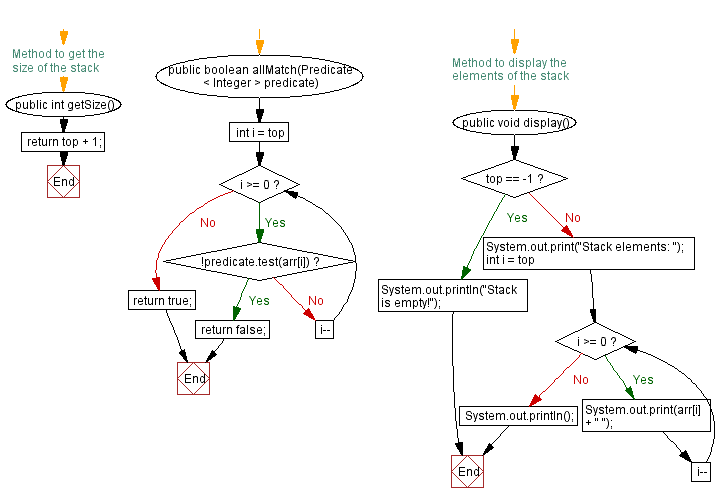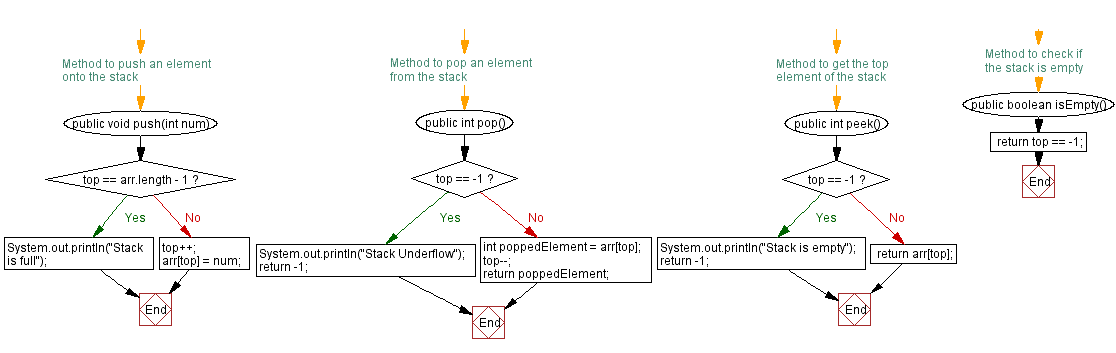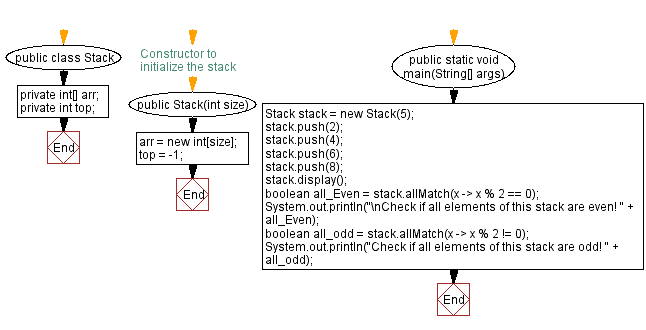# Java Exercises: Verify all stack elements satisfy a condition

## Java Stack: Exercise-26 with Solution

Write a Java program that implements a stack and checks if all elements of the stack satisfy a condition.

Sample Solution:

Java Code:

``````import java.util.*;
import java.util.function.Predicate;
public class Stack {
private int[] arr;
private int top;

// Constructor to initialize the stack
public Stack(int size) {
arr = new int[size];
top = -1;
}

// Method to push an element onto the stack
public void push(int num) {
if (top == arr.length - 1) {
System.out.println("Stack is full");
} else {
top++;
arr[top] = num;
}
}

// Method to pop an element from the stack
public int pop() {
if (top == -1) {
System.out.println("Stack Underflow");
return -1;
} else {
int poppedElement = arr[top];
top--;
return poppedElement;
}
}

// Method to get the top element of the stack
public int peek() {
if (top == -1) {
System.out.println("Stack is empty");
return -1;
} else {
return arr[top];
}
}

// Method to check if the stack is empty
public boolean isEmpty() {
}

// Method to get the size of the stack
public int getSize() {
}

public boolean allMatch(Predicate < Integer > predicate) {
for (int i = top; i >= 0; i--) {
if (!predicate.test(arr[i])) {
return false;
}
}
return true;
}

// Method to display the elements of the stack
public void display() {
if (top == -1) {
System.out.println("Stack is empty!");
} else {
System.out.print("Stack elements: ");
for (int i = top; i >= 0; i--) {
System.out.print(arr[i] + " ");
}
System.out.println();
}
}

public static void main(String[] args) {
Stack stack = new Stack(5);
stack.push(2);
stack.push(4);
stack.push(6);
stack.push(8);
stack.display();
boolean all_Even = stack.allMatch(x -> x % 2 == 0);
System.out.println("\nCheck if all elements of this stack are even! " + all_Even);
boolean all_odd = stack.allMatch(x -> x % 2 != 0);
System.out.println("Check if all elements of this stack are odd! " + all_odd);
}
}
```
```

Sample Output:

```Stack elements: 8 6 4 2

Check if all elements of this stack are even! true
Check if all elements of this stack are odd! false
```

Flowchart:Live Demo:

Java Code Editor:

Improve this sample solution and post your code through Disqus

Java Stack Previous: Symmetric difference of two stacks.
Java Stack Exercises Next: Verify at least one element satisfy a condition.

What is the difficulty level of this exercise?

Test your Programming skills with w3resource's quiz.

﻿